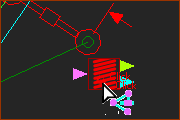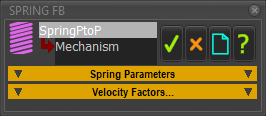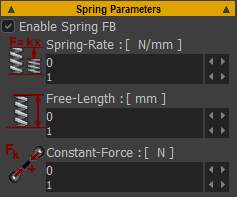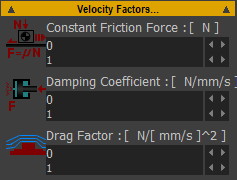﻿ MechDesigner Reference & User Interface > Dialog-Boxes > Dialog: Function-Block: Spring

# Dialog: Function-Block: Spring

Navigation:  MechDesigner Reference & User Interface > Dialog-Boxes >

# Dialog: Function-Block: Spring

## Spring FB

Use a Spring FB to apply a force between two Points. The name we use for the two Points is Anchor-Points.

Machine Settings > Engineering Units

Configure Power Source

#### How to open the Spring FB dialog-boxIn Mechanism-Editor:

 1.Double-click the Spring-FB in the graphic-area - or - 1.

Note: It is not necessary to connect a wire to the input-connector or from the output-connectors of the Spring FB. The input and output-connectors provide extra functionality - see Here

The Spring dialog-box is now open.

### Spring dialog-boxSpring ParametersCheck-box to enable the Spring FB.

Spring-Rate:

 Force Contribution: = Spring-Rate × | Spring-Length – Free-Length |

Spring-Length is the distance between the two anchor-points.

The Force always tries to move the anchor-point to the Free-Length (They cannot, of course).

Free-Length:

 The natural length of the Spring, when it is not joined to the Anchor-Points.

Constant-Force:

 A positive force will PULL Anchor-Points together (o>>> + <<>>o)

Velocity ParametersCoulomb Friction Force: (Ff = μ.Fn )

 A Constant Force that is opposite to the direction of the motion.

This parameter is intended to be similar to a Friction Force. However, it is not Friction Force, because the Coulomb Friction-Force parameter is not a function of the Normal Force.

Viscous Coefficient: (Fv α V ( N/(mm/s) )

 A Force that is proportional to the relative velocity between the Points. The Force is opposite to the direction of motion.

E.g. If the Damping Coefficient = 4 and the Velocity is +100mm/s, (anchor points moving away from each other), The force pulling the points together is a force of 400N.

Drag Factor: (Fd α V2) ( N/((mm/s)2 ) )

 A Force that is proportional to square of the relative velocity between the Points. For example, air resistance. The Force is opposite to the direction of motion.

E.g. If the Drag Factor = 4, and the Velocity = +100mm/s (anchor-points are moving apart), then the force pulling the anchor-points together is a force of 40000N.

#### Input-Connector and Output-Connectors

 Input-Connector Connect a wire to the input-connector to add a force-function between the Spring's Anchor-Points. The units of the data at the input-connector must be Newtons. Use a Math FB to convert the data-type of the Motion data-type to the Force data-type. For example, the function might be designed as a motion. In which case, you would need to connect the output of a Motion FB , which has units of mm or degrees to a Math FB to convert the units to Newtons. And then connect the output to the Spring-FB Note 1: Units inside the Math FB become SI units. To convert 100mm at the input to a Math FB to 100N, you must: 1.Change the Output Data Type to Force (N) AND 2.Multiply the input-value by 1000 to convert 0.1m to 100N TOP output-connector Dimension, Velocity and Acceleration relating to the distance between the Spring's anchor-points. Bottom output-connector Total Applied-Force between the Spring's anchor-points. oTotal Applied-Force = Force at the Input-connector + Force that results from the parameters as set in the Spring FB dialog-box or oDriving-Force = Linear Motive Force, if you configure the Spring FB as a Linear Motor -